• Machine Learning and Artificial Intelligence have become the new buzz words in the tech world; literally, everyone seems to have realized how important this field of study is.

A data scientist would agree that you can barely do without using a Jupyter notebook at some point in time, well, if not every time. A wide range of AI/ML engineers has adopted the use of Jupyter Notebook as a tool they use to write and test the algorithms/models.

But what is Jupyter? And why is it referred to as a Notebook?

According to Wikipedia, a notebook is a book or binder of paper of pages, often ruled, used for many purposes such as recording notes or memoranda, writing, drawing or scrap booking.

So basically, we could say a notebook is used to express a particular context, idea, or knowledge using text, diagrams, drawings, pictures, equations, tables, or even charts.

Why then is Jupyter referred to as a notebook?

Because it does exactly what the above stated does! It is used to draft out documents, codes, texts, pictures, equations, draft charts, and visualizations and even draw tables.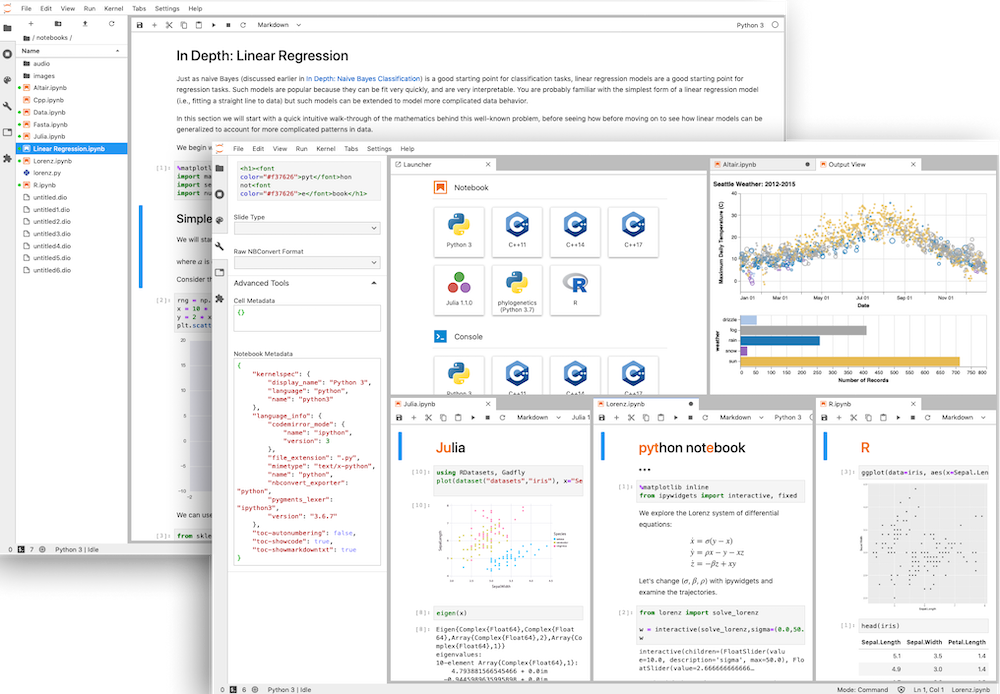## What is Jupyter Notebook?

The Jupyter Notebook is an open-source web application that allows you to create and share documents that contain live code, equations, visualizations, and narrative text. It uses include data cleaning and transformation, numerical simulation, statistical modeling, data visualization, machine learning, and much more.

Most often, the Jupyter Notebook is used in a Python environment. They have very interactive outputs and can be easily shareable, just like a regular notebook.

## What can Jupyter Notebook be used for?

Writing multiple languages.

The Jupyter system supports over 100 programming languages (called “kernels” in the Jupyter ecosystem), including Python, Java, R, Julia, Matlab, Octave, Scheme, Processing, Scala, and many more. You can share the code written in Notebook with others.

Here are a few languages that can be written in Jupyter notebook.

### Python

Of all languages that can be written with Jupyter, python is the most popular with the notebook. Almost everyone that writes code within the Jupyter environment writes the Python. By default, Jupyter supports Python in their environment without the use of special magic commands.

def hello_world():
print("Hello world!!!")
hello_world()

And, the output would be:

Hello world!!!

### JavaScript

JavaScript popularly known for the web and can also be written in Jupyter. Unlike Python, JavaScript isn’t supported by default. You have to use a certain special command to tell the cell you’re running it in that this is a JavaScript code. These commands are often called magic commands. for JavaScript, the command is %%javascript.

There is also a limit to what JavaScript code you could run in Jupyter Notebook, unlike python.

%%javascript
const text = "hello world"
alert(text)

### Java

It allows integrating additional “kernels” – languages. Such a kernel can be installed by following the set of installation instructions here. After installing, run the following command in your Jupyter terminal if on Linux.

jupyter console --kernel=java
Jupyter console 5.1.0
Java 9.0.4+11 :: IJava kernel 1.1.0-SNAPSHOT
Protocol v5.0 implementation by jupyter-jvm-basekernel 2.2.1-SNAPSHOT
In :

### Matlab

Matlab is a high-performance language for technical computing; It integrates computation, visualization, and programming in an easy-to-use environment where problems and solutions are expressed in familiar mathematical notation.

To use Matlab in Jupyter Notebook, you first have to install Jupyter-Matlab. The first thing we need to do is to create a virtual environment.

• Open your Jupyter prompt on windows or just your terminal on Linux and type in the following command
conda create -vv -n jmatlab python=3.5 jupyter
• Make sure you remain in this terminal, then type the code
source activate jmatlab
• Then install Matlab kernel for Python
pip install matlab_kernal
python -m matlab_kernel install
• Check if the kernel is properly installed
jupyter kernelspec list
• Find your MATLAB directory.  “/Applications/MATLAB_R2017a.app”.
• Go to the “extern/engines/python” subdirectory and install the Python engine.
cd “/Applications/MATLAB_R2017a.app/extern/engines/python”
python setup.py install
• Start Jupyter notebook
cd your_working_directory
jupyter notebook

Once launched, there should now be an option for both Matlab and python.

### Markdowns

Jupyter notebook comes in handy when it comes to writing markdown, and this can be very useful when you want to give a verbose or well-detailed explanation of a piece of code, write documentation, or a dictionary for a particular dataset.
Type the below code in a notebook.

* [Pandas](#pandas),
Used for data analysis
* [Numpy](#numpy),
Used for numerical analysis
* [Matplotlib](#matplotlib),
Used for data visualizations

The output should be as follows;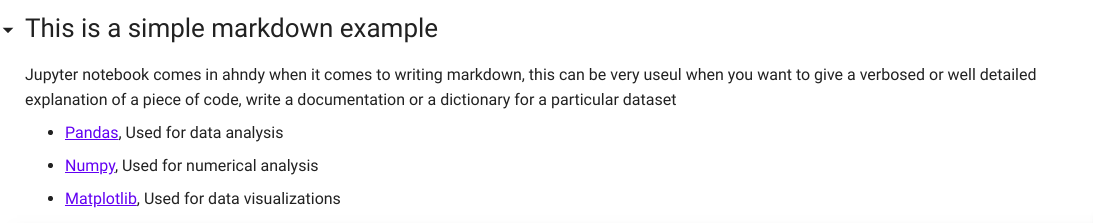### Bash Scripts

Jupyter Notebooks allows the use of bash script by using the %%bash magic command.

To test, let’s create a folder in your current working directory. Type the following code in a Notebook cell.

%%bash
mkdir Test_Folder

Run the code, now check your working directory by typing the code

%%bash
ls

You will see that the folder Test_Folderhas been added to it. You can also navigate to the folder physically to check.

### Data Visualization

With the use of Python libraries like matplotlib, you can run and display data visualizations right in your browser.

Let’s try to make a very basic visualization using matplotlib.

We would first import the library

from matplotlib import pyplot as plt
%matplotlib inline

Then type in the following codes

x = [1, 2, 3, 4, 5, 6, 7, 8, 9]
y = [11, 12, 13, 14, 15, 16, 17, 18, 19]
plt.plot(x, y)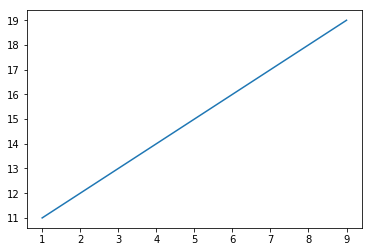Even more intriguing is we could do 3d visualizations!!
We first need to import the 3d visualization library

from mpl_toolkits import mplot3d
import numpy as np

Then make a 3d projection

fig = plt.figure()
ax = plt.axes(projection='3d')

Our output should look like thisNow, run the following scripts.

def f(x, y):
return np.sin(np.sqrt(x ** 2 + y ** 2))

x = np.linspace(-6, 6, 30)
y = np.linspace(-6, 6, 30)
X, Y = np.meshgrid(x, y)
Z = f(X, Y)

ax = plt.axes(projection='3d')
ax.plot_surface(X, Y, Z, rstride=1, cstride=1,
cmap='viridis', edgecolor='none')
ax.set_title('surface');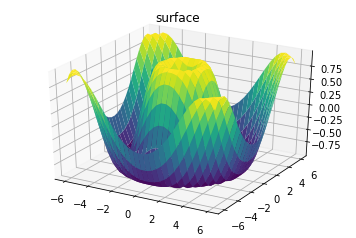### Mathematical And Scientific Notations

We can use tools like Latex right inside our Jupyter Notebook type mathematical and scientific equations.

LaTeX is a high-quality typesetting system; it includes features designed for the production of technical and scientific documentation. You can learn more about latex here here. Let’s try to run some simple LaTex codes.
Type in the following LaTex commands

## $J(\theta_0) = \frac{1}{2m}\sum_{i=0}^{m} (h_\theta(x^{(i)}) - y^{(i)})^2$

The output should be of this sort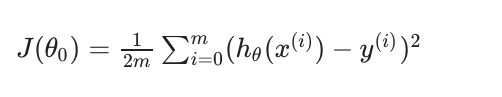Conclusion

This article just scratches the surface of what could be achieved with the use of Jupyter notebooks. You can find most of the examples in this article in this Jupyter notebook I created here on collaboratory